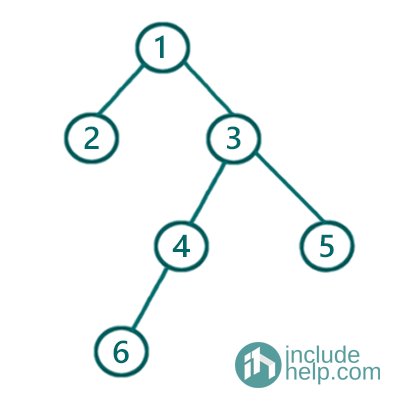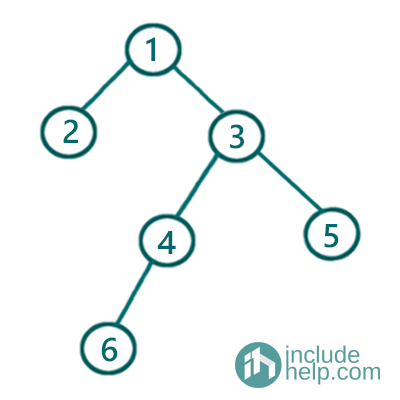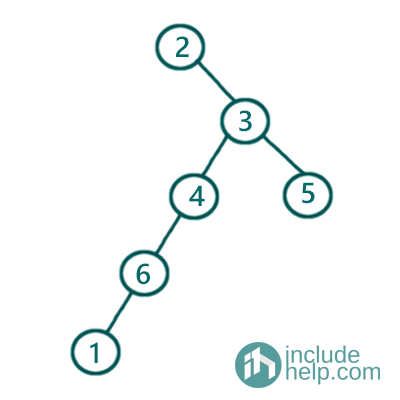# Checking if all three traversals inorder, preorder & postorder are of same binary tree or not

In this article, we are going to see, where all three given traversals are of the same tree or not.
Submitted by Radib Kar, on August 07, 2020

In this article, we are going to see If the given three traversals preorder, postorder & inorder are of the same tree or not.

In the article on Traversal from which we can construct the tree, inorder traversal, preorder traversal, we saw that to construct a tree from the traversals we need the inorder traversal and another traversal from which can be any out of preorder, postorder or level-order. Now, here we are given three traversals and we need to check whether all traversals are of the same tree or not. So, to check that we need to first construct the tree from either of

1. Inorder & preorder
2. Inorder & postorder

Case 1 (Approach 1- Construct tree from preorder & inorder traversal and check with postorder traversal)

If we construct the tree from its inorder & preorder traversals then after constructing we need to check the postorder traversal of the constructed tree along with the given postorder traversal. To check how we can construct a unique tree from its inorder & preorder traversal, please follow my article on How to construct a tree from preorder & inorder traversal.

For example, say the given traversals are:

Preorder traversal: [1, 2, 3, 4, 6, 5]
Inorder traversal: [2, 1, 6, 4, 3, 5]
Postorder traversal: [2, 6, 4, 5, 3, 1]

Then the tree constructed from the preorder & Inorder traversal is:Figure 1: Constructed tree from the above traversals

Please follow my tutorial on constructing a unique tree from its preorder & inorder traversal, to check how it has been constructed step by step.

So, now let's derive the postorder traversal of the above-constructed tree> To follow how to traverse postorder, please check my tutorial on postorder traversal.

So the postorder traversal of the above tree is [2, 6, 4, 5, 3, 1] which exactly matches the given postorder traversal. Thus, we can infer that all three traversals given belong to the same tree.

Now, if we check another example, say, the traversals given are below:

Preorder traversal: [1, 2, 3, 4, 6, 5]
Inorder traversal: [2, 1, 6, 4, 3, 5]
Postorder traversal: [1, 6, 4, 5, 3, 2]

Then obviously, the tree would remain same and so the postorder traversal of the constructed tree, but the postorder traversal of the constructed tree([2, 6, 4, 5, 3, 1]) doesn't match with the given postorder traversal([1, 6, 4, 5, 3, 2]). Hence, here all three traversals are not of the same tree.

Below is the Implementation of the above discussion.

C++ Implementation:

```#include <bits/stdc++.h>
using namespace std;

class TreeNode {
public:
int val;
TreeNode* left;
TreeNode* right;

TreeNode(int v)
{
val = v;
left = NULL;
right = NULL;
}
};

//searching the root value in the inorder
//traversal stored vector
int search(vector<int> a, int k)
{
for (int i = 0; i < a.size(); i++)
if (a[i] == k)
return i;

return -1;
}

TreeNode* buildTreeRecur(vector<int>& preorder, vector<int>& inorder, int& index, int left, int right)
{
if (index == preorder.size())
return NULL;
//base case to return child of leaf nodes NULL
if (left > right)
return NULL;
/*
preorder[index] is the root
search that in the inorder vector, say position found is in_index
then [left,k-1] will have the left subtree for the current root
[k+1, right] will have traversal for right subtree for the current root
recursively build the subtrees
*/
int in_index = search(inorder, preorder[index]);
int k = preorder[index];
index++;
//root created
TreeNode* root = new TreeNode(k);
//recursively building the subtree
root->left = buildTreeRecur(preorder, inorder, index, left, in_index - 1);
root->right = buildTreeRecur(preorder, inorder, index, in_index + 1, right);
return root;
}

TreeNode* buildTree(vector<int>& preorder, vector<int>& inorder)
{
int index = 0;
int n = preorder.size();
return buildTreeRecur(preorder, inorder, index, 0, n - 1);
}

void postorder_traversal(TreeNode* root, vector<int>& postorder_from_constructed_tree)
{
if (!root)
return;

postorder_traversal(root->left, postorder_from_constructed_tree);
postorder_traversal(root->right, postorder_from_constructed_tree);
postorder_from_constructed_tree.push_back(root->val);
}

int main()
{
cout << "Enter Number of nodes in the tree\n";
int n;
cin >> n;

vector<int> preorder(n);

cout << "Enter the preorder traversal of the tree\n";
for (int i = 0; i < n; i++)
cin >> preorder[i];

vector<int> inorder(n);
cout << "Enter the inorder traversal of the tree\n";
for (int i = 0; i < n; i++)
cin >> inorder[i];

vector<int> postorder(n);
cout << "Enter the postorder traversal of the tree\n";
for (int i = 0; i < n; i++)
cin >> postorder[i];

TreeNode* root = buildTree(preorder, inorder);

vector<int> postorder_from_constructed_tree;

postorder_traversal(root, postorder_from_constructed_tree);

if (postorder.size() != postorder_from_constructed_tree.size()) {
cout << "All the three traversals are not of the same tree\n";
}
else {
bool flag = true;
for (int i = 0; i < postorder.size(); i++) {
if (postorder[i] != postorder_from_constructed_tree[i]) {
flag = false;
break;
}
}
if (flag) {
cout << "All the three traversals are of the same tree\n";
}
else
cout << "All the three traversals are not of the same tree\n";
}

return 0;
}
```

Output:

```RUN 1:
Enter Number of nodes in the tree
6
Enter the preorder traversal of the tree
1 2 3 4 6 5
Enter the inorder traversal of the tree
2 1 6 4 3 5
Enter the postorder traversal of the tree
2 6 4 5 3 1
All the three traversals are of the same tree

RUN 2:
Enter Number of nodes in the tree
6
Enter the preorder traversal of the tree
1 2 3 4 6 5
Enter the inorder traversal of the tree
2 1 6 4 3 5
Enter the postorder traversal of the tree
1 6 4 5 3 2
All the three traversals are not of the same tree
```

Case 2 (Approach 2- Construct tree from postorder & inorder traversal and check with preorder traversal)

As we know that we can also construct a unique tree from its postorder & inorder traversal. So, if we construct the tree from its inorder & postorder traversals, then after constructing we need to check the preorder traversal of the constructed tree along with the given preorder traversal. To check how we can construct a unique tree from its inorder & postorder traversal, please follow my article on How to construct a tree from postorder & inorder traversal.

For example, say the given traversals are:

Preorder traversal: [1, 2, 3, 4, 6, 5]
Inorder traversal: [2, 1, 6, 4, 3, 5]
Postorder traversal: [2, 6, 4, 5, 3, 1]

Then the tree constructed from the postorder & Inorder traversal is:Figure 2: Constructed tree from the above traversals

Please follow my tutorial on constructing a unique tree from its inorder & postorder traversal, to check how it has been constructed step by step.

So, now let's derive the preorder traversal of the above-constructed tree. To follow how to traverse preorder, please check my tutorial on preorder traversal.

So the preorder traversal of the above tree is [1, 2, 3, 4, 6, 5] which exactly matches with the given preorder traversal. Thus, we can infer that all three traversals given belong to the same tree.

Now, if we check another example, say, the traversals given are below:

Preorder traversal: [1, 2, 3, 4, 6, 5]

Inorder traversal: [2, 1, 6, 4, 3, 5]

Postorder traversal: [1, 6, 4, 5, 3, 2]

Then obviously, the newly constructed tree would change. If we follow the steps shown in the tutorial on constructing a tree from its postorder & inorder traversal, we will be able to build a tree like below from the given postorder & inorder traversals.Figure 3: New constructed Tree

So, the preorder traversal for the above tree will be [2, 3, 4, 6, 1, 5] which is not same as the given preorder traversal [1, 2, 3, 4, 6, 5]. So, all three traversals are not of the same tree.

Below is the Implementation of the above discussion.

C++ Implementation:

```#include <bits/stdc++.h>
using namespace std;

// tree node is defined
class TreeNode {
public:
int val;
TreeNode* left;
TreeNode* right;
TreeNode(int data)
{
val = data;
left = NULL;
right = NULL;
}
};

//Searching the root position in the inorder traversal
int search(vector<int> a, int k)
{

for (int i = 0; i < a.size(); i++)
if (a[i] == k)
return i;

return -1;
}

TreeNode* buildTreeRecur(int& index, int left, int right, vector<int>& inorder, vector<int>& postorder)
{
//base case
if (left > right)
return NULL;
if (index < 0)
return NULL;

/*
postorder[index] is the root
search that in the inorder vector, say position found is pivot
then [left,pivot-1] will have the left subtree for the current root
[pivot+1, right] will have traversal for right subtree for the current root
recursively build the subtrees
*/

//search the root position in the inorder traversal
int pivot = search(inorder, postorder[index]);
//decrement index for next root
index--;
//create the root
TreeNode* root = new TreeNode(inorder[pivot]);
//build the right subtree recursively
root->right = buildTreeRecur(index, pivot + 1, right, inorder, postorder);
//build the right subtree recursively
root->left = buildTreeRecur(index, left, pivot - 1, inorder, postorder);

return root;
}
TreeNode* buildTree(vector<int>& inorder, vector<int>& postorder)
{
int index = postorder.size() - 1;
int l = 0, r = inorder.size() - 1;
return buildTreeRecur(index, l, r, inorder, postorder);
}

void preorder_traversal(TreeNode* root, vector<int>& preorder_from_constructed_tree)
{
if (!root)
return;
preorder_from_constructed_tree.push_back(root->val);
preorder_traversal(root->left, preorder_from_constructed_tree);
preorder_traversal(root->right, preorder_from_constructed_tree);
}

int main()
{
cout << "Enter Number of nodes in the tree\n";
int n;
cin >> n;

vector<int> preorder(n);

cout << "Enter the preorder traversal of the tree\n";
for (int i = 0; i < n; i++)
cin >> preorder[i];

vector<int> inorder(n);

cout << "Enter the inorder traversal of the tree\n";
for (int i = 0; i < n; i++)
cin >> inorder[i];

vector<int> postorder(n);

cout << "Enter the postorder traversal of the tree\n";
for (int i = 0; i < n; i++)
cin >> postorder[i];

TreeNode* root = buildTree(inorder, postorder);

vector<int> preorder_from_constructed_tree;

preorder_traversal(root, preorder_from_constructed_tree);

//checking whether given preorder traversal & the constructed
//preorder traversal is the same or not
if (preorder.size() != preorder_from_constructed_tree.size()) {
cout << "All the three traversals are not of the same tree\n";
}
else {
bool flag = true;
for (int i = 0; i < preorder.size(); i++) {
if (preorder[i] != preorder_from_constructed_tree[i]) {
flag = false;
break;
}
}
if (flag) {
cout << "All the three traversals are of the same tree\n";
}
else
cout << "All the three traversals are not of the same tree\n";
}

return 0;
}
```

Output:

```RUN 1:
Enter Number of nodes in the tree
6
Enter the preorder traversal of the tree
1 2 3 4 6 5
Enter the inorder traversal of the tree
2 1 6 4 3 5
Enter the postorder traversal of the tree
2 6 4 5 3 1
All the three traversals are of the same tree

RUN 2:
Enter Number of nodes in the tree
6
Enter the preorder traversal of the tree
1 2 3 4 6 5
Enter the inorder traversal of the tree
2 1 6 4 3 5
Enter the postorder traversal of the tree
1 6 4 5 3 2
All the three traversals are not of the same tree
```

What's New

Top Interview Coding Problems/Challenges!

Languages: » C » C++ » C++ STL » Java » Data Structure » C#.Net » Android » Kotlin » SQL
Web Technologies: » PHP » Python » JavaScript » CSS » Ajax » Node.js » Web programming/HTML
Solved programs: » C » C++ » DS » Java » C#
Aptitude que. & ans.: » C » C++ » Java » DBMS
Interview que. & ans.: » C » Embedded C » Java » SEO » HR
CS Subjects: » CS Basics » O.S. » Networks » DBMS » Embedded Systems » Cloud Computing
» Machine learning » CS Organizations » Linux » DOS
More: » Articles » Puzzles » News/Updates